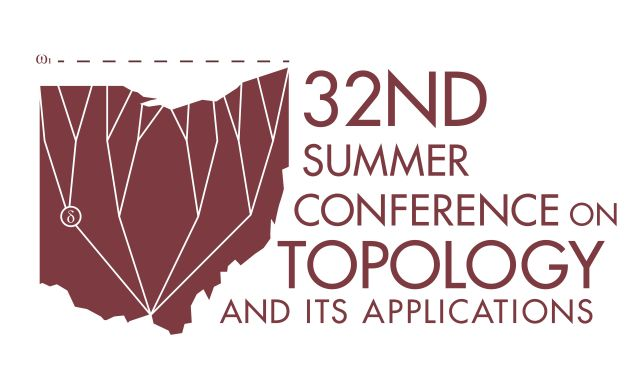#### Document Type

Topology + Algebra and Analysis

6-2017

#### Publication Source

32nd Summer Conference on Topology and Its Applications

#### Abstract

For a limit ordinal λ, let (Aα)α < λ be a system of topological algebras (e.g., groups or vector spaces) with bonding maps that are embeddings of topological algebras, and put A = ∪α < λ Aα. Let (A, T) and (A, A) denote the direct limit (colimit) of the system in the category of topological spaces and topological algebras, respectively. One always has TA, but the inclusion may be strict; however, if the tightness of A is smaller than the cofinality of λ, then A=T.

In 1988, Tkachenko proved that the free topological group F(X) is sequential when Xn is sequential, countably compact, and normal for every n. In particular, F(ω1) is sequential.

In this talk, we show that under the same conditions, the free topological vector space V(X) is sequential, and thus countably tight. Consequently, F(ω1) = colimα < ω1 F(α) and V(ω1) = colimα < ω1 V(α) not only as topological algebras, but also as topological spaces.

This document is available for download with the permission of the presenting author and the organizers of the conference. Permission documentation is on file.

Technological limitations may prevent some mathematical symbols and functions from displaying correctly in this record’s metadata fields. Please refer to the attached PDF for the correct display.

COinS